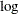Displayed Output for Classical Analysis

For each model, PROC LIFEREG displays the following.

### Model Information

The "Model Information" table displays the two-level name of the input data set, the distribution name, and the name and label of the dependent variable; the name and label of the censor indicator variable, for right-censored data; if you specify the WEIGHT statement, the name and label of the weight variable; and the maximum value of the log likelihood.

### Number of Observations

The "Number of Observations" table displays the number of observations read from the input data set, and the number of observations used in the analysis.

### Class Level Information

The "Class Level Information" table displays the levels of classification variables if you specify a CLASS statement.

### Fit Statistics

The "Fit Statistics" table displays the negative of twice the log likelihood, Akaike’s information criterion (AIC), the corrected Akaike’s information criterion (AICC), and the Bayesian information criterion (BIC). If the specified distribution is Weibull, lognormal, log-logistic, or gamma, the fit criteria are based on the log likelihood for the log of the response, rather than for the response on the original scale.

### Fit Statistics (Unlogged Response)

If the specified distribution is Weibull, lognormal, log-logistic, or gamma, the "Fit Statistics (Unlogged Response)" table displays fit criteria that are based on the log likelihood for the response on the original, rather than, scale. The negative of twice the log likelihood, Akaike’s information criterion (AIC), the corrected Akaike’s information criterion (AICC), and the Bayesian information criterion (BIC) are displayed.

### Type III Analysis of Effects

The "Type III Analysis of Effects" table displays, for each effect in the model, the effect name, the degrees of freedom associated with the type III contrast for the effect, the chi-square statistic for the contrast, and the p-value for the statistic.

### Analysis of Maximum Likelihood Parameter Estimates

The "Analysis of Maximum Likelihood Parameter Estimates" table displays the parameter name, the degrees of freedom for each parameter, the maximum likelihood estimate of each parameter, the estimated standard error of the parameter estimator, confidence limits for each parameter, a chi-square statistic for testing whether the parameter is zero, and the associated p-value for the statistic.

### Lagrange Multiplier Statistics

If there are constrained parameters in the model, such as the scale or intercept, then the "Lagrange Multiplier Statistics" table displays a Lagrange multiplier test for the constraint.